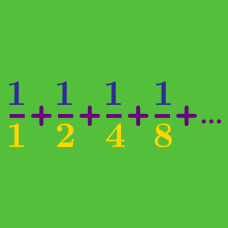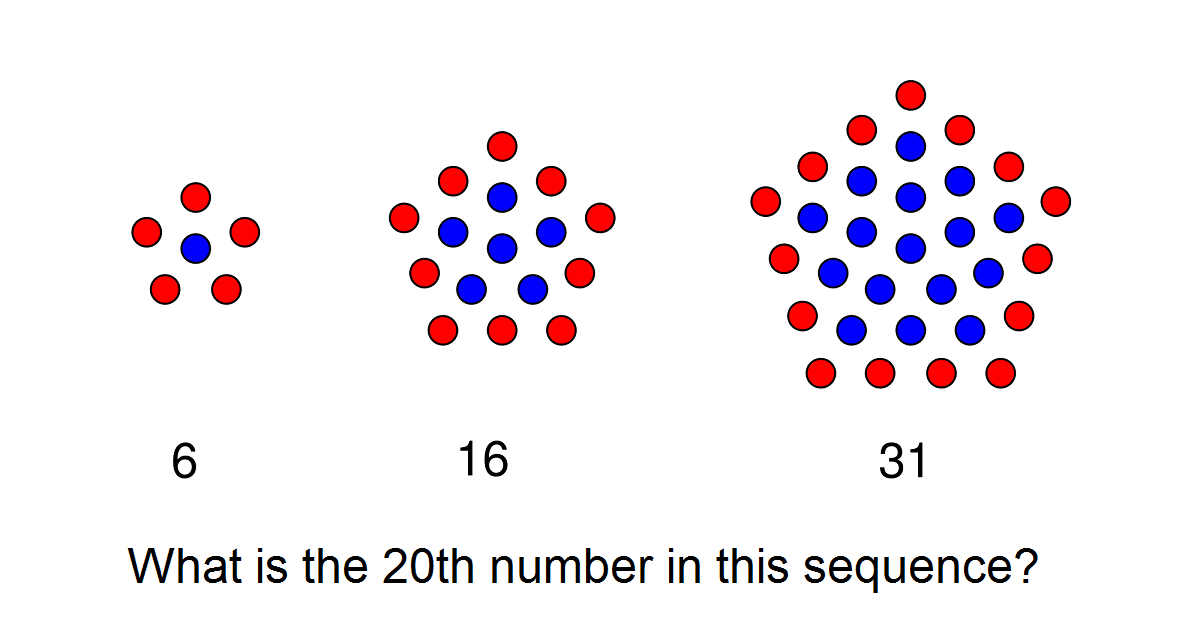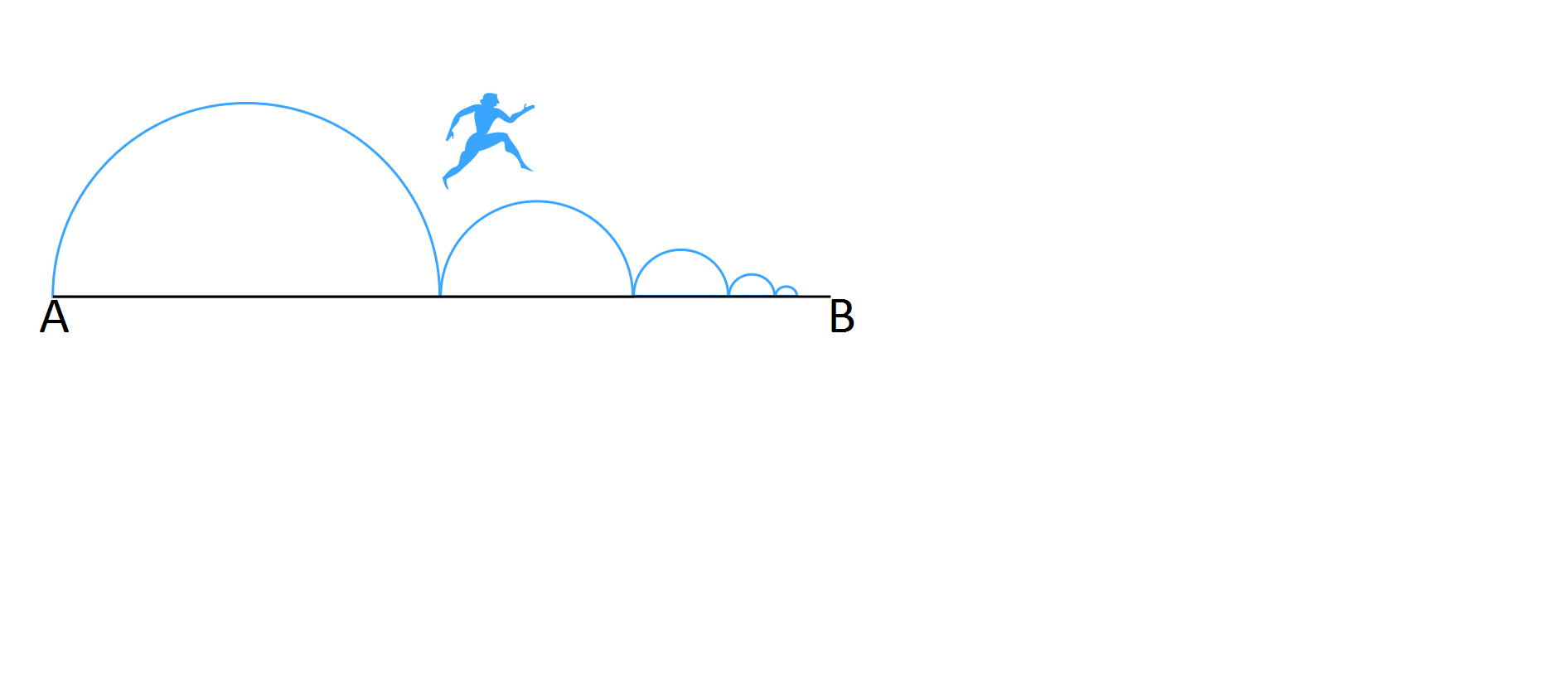Calculus

# Finite Series

Evaluate $1 + 2 + 3 + \cdots + 98 + 99 + 100 = \sum_{i=1}^{100} i.$

Let $S_n = \sum_{k=1}^{n} k^2.$

Which of the following is not equal to $S_6$?These numbers are called centered pentagonal numbers. A finite series can be used to find the answer.

Let $\displaystyle S_n = \sum_{k=1}^{n} (-1)^{k^2 + k}$. Then $S_n = \,$?The distance from A to B is 10 kilometers. Starting at A, Zeno repeats the following step:

Iterative Step: Walk halfway to B; this point is the starting point for the next iteration.

How many kilometers will Zeno have walked after performing the iterative step 100 times?

×